## Friday, November 17, 2006

### Lodovico Ferrari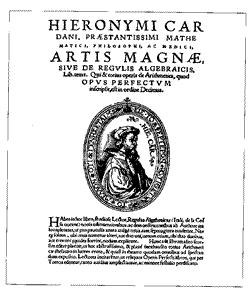Lodovico Ferrari was born on February 2, 1522. His father died when he was very young so he lived with his uncle Vincent Ferrari. The mathematician Gerolamo Cardano was looking for a servant. Vincent's son Luke took the job but decided he didn't like it and left. Cardano then brought the matter up with Vincent. Vincent sent Lodovico to Cardarno in Luke's place.

On November 30, 1536, fourteen-year old Ferrari became Cardano's servant. Over time, Cardano was very impressed by Ferrari's intelligence and soon began to teach him mathematics. Ferrari began to collaborate with Cardano on his manuscripts and by the time that Ferrari was 18, he began to lecture on mathematics.

In 1540, Ferrari came up with the general solution for the quartic equation. Cardano would later publish this method in his famous book Ars Magna. In 1541, when Cardano resigned his post in mathematics in Milan, Ferrari, then 20, was able to succeed him.

Cardano had earlier solved the general cubic equation which extended a method he had learned from Nicolo Tartaglia. Cardano had promised Tartaglia that he would not publish the method until after Tartaglia published. It was this agreement that held up the publishing of Ars Magna. Cardano was very anxious to publish the work and when he found that Tartaglia's method was based on the method of Scipione del Ferro, he decided in 1545 to publish the work despite his promise to Tartaglia.

Ferrari supported Cardano in the resulting controversy and challenged Tartaglia to a public debate. Ferrari was not yet a well known mathematician so Tartaglia refused to debate him and insisted on challenging Cardano. Nothing happened for three years. Then, in 1548, Tartaglia was offered an important position in mathematics on the condition that he publicly debated Ferrari. By this time, the controversy between the two had become very public and there was a strong popular interest in this mathematical challenge.

The open debate occurred on August 10, 1548 in Milan. It occurred in front of a large crowd. By this time, Ferrari had mastered both the cubic and quartic equations. In the course of the discussion, it was clear that Ferrari was the master of the two and before the contest ended, Tartaglia decided to withdraw. In this way, Ferrari's reputation was greatly established as one of Italy's top mathematicians.

From this point on, Ferrari accepted a series of well paid positions and in 1565, he moved to Bologna where he stayed with his recently widowed half-sister and worked as a professor to the University of Bologna. Within one year, he died very suddenly. It was rumored that he had been poisoned by his half sister who sought to inherit his great wealth. Indeed, after his death, she received all of his estate. Then, she remarried and her husband absconded with all her money.

References

## Thursday, November 16, 2006

### Girolamo Cardano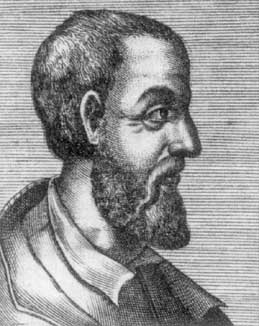Girolamo Cardano's father Fazio Cardano, a lawyer by profession, had a deep interest in mathematics. He had developed a reputation as an expert and lectured on geometry at the University of Pavia. It was said that Leonardo da Vinci once consulted with him on mathematics.

Chiara Cardano, Girolamo's mother, was a widow with three children when Fazio met her. This was a time of the plague and when Chiara was close to giving birth, she traveled to Pavia to avoid the plague which had hit Milan where she lived. She would later learn that her three children had caught the plague and died. When Cardano was born, his parents were not married.

Cardano was born on September 24, 1501. He was a sickly child. As he grew older, he worked as an assistant to his father where he learned mathematics. Despite his interest in mathematics, when he entered the University of Pavia, he decided to study medicine.

This was a time of instability and war in Italy and when the University of Pavia was temporarily shut down, Cardano transferred to the University of Padua.

It was at this same time that his father died. Cardano received a small inheritance which he quickly spent. He began to rely on gambling as a source of income.

In 1525, Cardano graduated from the university and sought to become a member of Milan's College of Physicians. His application was rejected because of his illegitimate birth.

Cardano tried to set up a medical practice in a small town. There, he met his wife, Lucia but was unable to succeed as a physician. He applied again to the College of Physicians in Milan and again was rejected. By 1533, gambling was taking up his time and money. He pawned his wife's jewelry at this time and found himself in the poorhouse.

Cardano's fortune changed when he managed to get his father's old position as lecturer in mathematics. At the same time, his medical practice began to take off even though he had not been accepted by the College of Physicians. In 1536, he wrote a book critical of the College of Physicians. Despite this, his reputation and influence grew. Using his growing social connections, he was able to become a member of College of Physicians in 1538.

In 1539, he heard about the mathematician Tartaglia who had discovered a method to solve certain forms of cubic equations. He convinced Tartaglia to reveal the details of his method which Tartaglia did on the condition that Cardano wouldn't publish the method unless Tartaglia published it first.

Over the next six years, Cardano began to study cubic and quartic equations. It was at this time that he was able to come up with a general solution for the cubic equations using radicals. This was a significant advance over the method of Tartaglia.

Later, Cardano's assistant, Lodovico Ferrari came up with a general solution of quartic equations using radicals. In 1545, Cardano published his very important book Ars Magna in which he discussed the general solution for the cubic equation and the quartic equation. The book was a best seller and established Cardano's reputation as a great mathematician. There is much that has been written about the dispute between Cardano and Tartaglia so I will not write about it here. (See the references for more details.)

In 1546, his wife Lucia died. By this time, he had become rector of the College of Physicians in Milan. He once traveled to Scotland in order to provide a cure for Archbishop of St. Andrews. The archbishop made a complete recovery. Cardano was now helping a very rich set of clients. He was a wealthy man and he became professor of medicine at Pavia University.

Cardano's eldest son was in an unhappy marriage. After years of unhappiness, he poisoned his wife and confessed to the murder. The judge ruled that his son's life could be spared if Cardano could settle with the wife's parents. Such a settlement did not occur and Cardano's son was executed on April 13, 1560.

Cardano now moved to Bologna where he became a professor of medicine. His other son had a terrible gambling problem and once, when in need of money, took large amounts of money from his father. The son was later banished from Bologna for the crime.

In 1570, Cardano was accused of being a heretic for providing a horoscope and for writing a book that praised the emperor Nero. It is believed that his son helped to raise the charges. Cardano was now imprisoned for several months. On his release, he traveled to Rome where he met with the Pope. Somehow, despite everything that had occurred, the pope granted him a pension.

Cardano predicted the date of his death and sure enough, he died on that day. It is said that he committed suicide. Although, he later made peace with his son, in his will, he left all of his belongings to his grandson.

References

## Wednesday, November 15, 2006

### Abu Ja'far Muhammad ibn Musa Al-Khwarizmi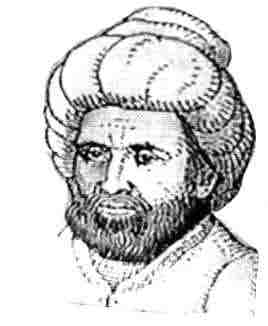Unfortunately, there are many details missing from our knowledge about Abu Ja'far Muhammad ibn Musa Al-Khwarizmi. He was born around 780 AD. It is unclear where he was born. His name Al-Khwarizmi may imply that he was from Khwarizm (Khiva) in Central Asia. Again, this may imply that his family is from Khwarizm but he himself was from Baghdad.

We do know that Al-Kwarizmi became a scholar at the House of Wisdom in Baghdad. His role was to translate books from Greek as well as write original works on mathematics, astronomy, and other topics. The House of Wisdom was an academy that had been set up by Al-Mamun who became caliph of the Arab Empire in 813 AD after defeating his brother. In addition to the House of Wisdom, Al-Mamun set up a great library and and several astronomy observatories. The library at this time was perhaps the most important collection of Greek and Byzantine books since the great library of Alexandria.

We know that Al-Khwarizmi dedicated two of his books to Caliph Al-Mamun. One of these books was on astronomy and the other book was the very famous text on algebra called Hisab al-jabr w'al-muqabala. It is from this book that we get the English work algebra (the Latin translation of the book was Liber algebrae et almucabala). This second book launched the rise of algebra in its ascendency over the geometry of the Greeks. Another of his books on arithmetic popularized what became known as Arabic numerals.

The long title of the book on algebra refers to two important operations. al-jabr ("completion") and al-muqabala ("balancing"). The book systematizes linear and quadratic equations and focuses on numbers (as opposed to geometric shapes) which include units (a number), roots (x), and squares (x2).

The book is written almost completely in words. Even numbers are written as words. Although Al-Khwarizmi popularized the Arabic numerals in another of his books, he does not use them here. He categorizes quadratic equations into six types:
• "squares" equal "roots" (ax2 = bx)
• "squares" equal number (ax2 = c)
• "roots" equal number (bx = c)
• "squares" and "root" equal number (ax2 + bx = c)
• "squares" and number equal "roots" (ax2 + c = bx)
• "roots" and number equal "square" (bx + c = ax2)
He then presents algebraic and geometric methods for solving each of these types.

Interestingly, his book does not include a the general solution for quadratic equations even though, it is implied in the solution for each of the problems in the book. This would be first published in 1145 by Abraham bar Hiyya Ha-Nasi.

Al-Khwarizmi's book on arithmetic was called Al-Khwarizmi on the Hindu Art of Reckoning. Unfortunately, all Arabic versions of this book have been lost. The only extent book is a Latin translation.

This book on arithmetic has given us the modern word "algorithm" and it is the place where the Hindu numerals are introduced to the West and the place where 0 is used as a number. This book consists of a systematization of the Indian numerals.

Al-Khwarizmi wrote many other books including books on astronomy, geography, calendars, sun dials, and many other topics. Unfortunately, it is very difficult to know how much of this content was original and how much was taken from other sources.

What is undeniable is the great importance of Al-Khwarizmi's work and the impact it had on the rise of algebra.

One scholar has written (see the MacTutor reference):
In the foremost rank of mathematicians of all time stands Al-Khwarizmi. He composed the oldest works on arithmetic and algebra. They were the principal source of mathematical knowledge for centuries to come in the East and the West. The work on arithmetic first introduced the Hindu numbers to Europe, as the very name algorism signifies; and the work on algebra ... gave the name to this important branch of mathematics in the European world...

References

## Tuesday, November 14, 2006

### Detour through Group Theory

To move ahead on the Kummer Class Formula, it is necessary to delve into Dirichlet Characters and L-Functions. Properly appreciating these ideas requires a solid understanding of cyclic groups.

I think that it makes sense at this point to take a break from Kummer's Class Formula and trace the development of group theory from the quadratic equation to Galois's famous proof.

Once I have gone into more detail about group theory and in particular, cyclic groups, I will resume the development of Kummer's Class Formula.

## Monday, November 13, 2006

### Derivation of the Kummer Class Formula: Step One

In the next series of blogs, I will show how the Kummer Class Formula is derived from the zeta function for cyclotomic integers. In a previous blog, I showed Kummer's proof that Fermat's Last Theorem was true for regular primes. The class formula is the method for determining whether a given prime is regular or not.

The content in today's blog is taken from Harold M. Edwards
Lemma 1:

If β is an fth root of unity and n=ef, then:

(x - yβ)*...*(x - yβn) = (xf - yf)e

Proof:

(1) Xf - 1 has f distinct roots by the Fundamental Theorem of Algebra (see here)

(2) This means that:

Xf - 1 = (X - 1)(X - β)(X - β2)*...*(X - βf-1)

(3) Since βf = 1, we have:

Xf - 1 = (X - β)(X - β2)*...*(X - βf-1)(X - βf)

(4) Let X = x/y, then we have:

(x/y)f - 1 = ([x/y] - β)([x/y] - β2)*...*([x/y] - βf)

(5) If we multiply both sides by yf, we get:

xf - yf = (x - βy)(x - β2y)*...*(x - βfy)

(6) Further, if we put both sides to the power of e, we get:

(xf - yf)e = [(x - βy)(x - β2y)*...*(x - βfy)]e =

= (x - βy)(x - β2y)*...*(x - βfy)*
(x - βf+1y)(x - βf+2)*...*(x - β2fy)*
...
(x - βf(e-1)+1)(x - βf(e-1)+2)*...*(x - βefy) =

= (x - βy)*x - β2y)*...*(βny)

QED

Theorem 2:Proof:

(1) Since N(P)=pf and N(α - 1)=λ (see here for pf and here for N(α-1)=λ) and since there are e prime divisors P that divide a prime p (see here), we have: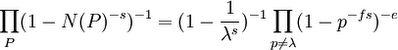(2) For each p, we can see that:

(1 - p-fs)-e = (1f - (p-s)f)-e

(3) Let γ be a primitive root mod λ

(4) For each p, let γj ≡ p (mod λ)

(5) Let βj be an fth root of unity such that j)f = 1 but for any integer i less than f, j)f ≠ 1.

(6) Using Lemma 1 above, we have:

(1f - (p-s)f)e = (1 - βjp-s)*...*(1 - p-sβjn) = ∏ (k=1, λ-1) (1 - βjkp-s) =

=∏ (k=0, λ-2) (1 - β
jkp-s)

(7) Using step #6, we have:

(1 - λ-s)-1 ∏ (p ≠ λ) (1 - p-fs)-e = (1 - λ-s)-1 ∏ (p ≠ λ) ∏ (k=0, λ-2) (1 - p-sβjk)-1

(8) From this step, the conclusion follows:QED

References

## Sunday, November 12, 2006

### The Zeta Function for Cyclotomic Integers

To get to the Kummer class formula, it is necessary to establish a zeta function for cyclotomic integers: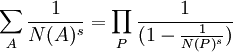where A ranges over the set of ideal numbers, P ranges over the set of prime divisors of ideal numbers, and N(X) is the norm for these ideal numbers [for review of ideal numbers, start here] and s is greater than 1.

The content in today's blog is taken from Harold M. Edwards' Fermat's Last Theorem: A Genetic Introduction to Algebraic Number Theory.

Theorem 1: For s greater than 1, ∏ (1 - N(P)-s)-1 converges absolutely

Proof:

(1) Let λ be a prime number such that αλ = 1 for any positive integer i less than λ, αi ≠ 1. [See here for review of cyclotomic integers and λ]

(2) N(P) = pf where p is a prime divisible by the prime divisor P and f is the exponent of p mod λ (or f=1 if p = λ) [See Lemma 1, here for proof N(P)=pf or here for f=1 if p=λ]

(3) N(P)-s = p-fs ≤ p-s

(4) Let e = (λ - 1)/f [See Lemma 1, here for proof that f divides λ-1] so that e ≤ λ - 1.

(5) We know from a previous result (see Lemma 1, here), that there are e prime divisors for a given prime p so that we know that:

∑ N(P) for a given P ≤ (λ - 1)∑ pf

(6) So that:

∑ N(P)-s ≤ (λ - 1) ∑ p-fs which is less than (λ - 1)∑ p-s which is less than (λ - 1)∑ n-s

(7) From a previous result (see Theorem 1, here), we know that ∑ n-s converges if s is greater than 1 so, we can conclude that ∑ N(P)-s necessarily converges.

(8) Since N(P)=pf is greater than 0, it follows that ∑ N(P)-s = ∑ abs(N(P)-s) and using Lemma 3, here, we can conclude that:

∏ (1 - abs(N(P)-s)) converges

(9) This gives us (see Definition 1, here) that ∏ (1 - N(P)-s) converges absolutely.

(10) Using the Reciprocal Law (see Lemma 6, here), we conclude that ∏ (1 - N(P)-s)-1 also converges absolutely.

QED

Lemma 2: For s greater than 1, ∑ N(A)-s ≤ ∏ (1 - N(P)-s)-1

Proof:

(1) Let A* be any set of A and S* be defined such that S* = ∑ (A ∈ A*) N(A)-s

(2) Let P* be the set of prime divisors that make up any of the A in A*. [See here for a review of prime divisors of an ideal number]

(3) For each P ∈ P*, we see that (1 - N(P)-s)-1 = 1 + N(P)-s + N(P2)-s + N(P3)-s + .... [ See Lemma 1, here]

(4) It is then clear that ∏ (1 - N(P)-s)-1 = the product of all of these geometric series which includes all A in A*.

(5) Since N(Pi) -s continues ad infinitum, there also necessarily exists N(Pi)-s that is not a divisor of any N(A) where A ∈ A* since A* is a finite set by assumption.

(6) So, any partial sum is less than the partial product.

(7) In this way, we can conclude that the infinite sum ∑ N(A) ≤ the infinite product ∏ (1 - N(P)-s)-1

QED

Corollary 2.1: ∑ N(A)-s converges absolutely.

Proof:

(1) By Lemma 2 above, ∑ N(A)-s ≤ ∏ (1 - N(P)-s)-1.

(2) Since ∏ (1 - N(P)-s)-1 converges (see Theorem 1 above), then ∑ N(A)-s necessarily converges too.

(3) Since all values N(A)-s are positive, it follows that ∑ N(A)-s converges absolutely. (see Definition 2, here)

QED

Theorem 3: ∑ N(A)-s = ∏ (1 - N(P)-s)-1

Proof:

(1) By Lemma 2 above, we have:

∑ N(A)-s ≤ ∏ (1 - N(P)-s)-1

(2) Let P* be the same set of prime divisors from Lemma 2 above.

(3) It is clear that ∏ (1 - N(P)-s)-1 includes as a sum a distinct set of ideal numbers but it does not include all ideal numbers since P* is a finite of P and there are an infinite number of primes p [See Theorem, here].

(4) Let A* be all the set of ideal numbers in the sum ∏ (1 - N(P)-s)-1 plus an ideal number A+ that is divisible by a prime divisor P that is not found in P*.

(5) It is clear that ∑ (A ∈ A*) N(A)-s) ≥ ∏ (P ∈ P*) (1 - N(P)-s)-1

(6) Combining step #5 with step #1 gives us that:

∑ N(A)-s = ∏ (1 - N(P)-s)-1

QED

References